Courses

# General P-V-T Behaviour of Real Fluids P-V Diagrams Civil Engineering (CE) Notes | EduRev

## Civil Engineering (CE) : General P-V-T Behaviour of Real Fluids P-V Diagrams Civil Engineering (CE) Notes | EduRev

The document General P-V-T Behaviour of Real Fluids P-V Diagrams Civil Engineering (CE) Notes | EduRev is a part of the Civil Engineering (CE) Course Thermodynamics.
All you need of Civil Engineering (CE) at this link: Civil Engineering (CE)

Volumetric Properties of Real Fluids

Fig. 2.1 represents the general pure component, real fluid phase behavior that typically obtains from experimental measurements. Consider first the fig. 2.1a. Let us take a substance at some temperature T1 and certain pressure P1 such that it is in a gaseous state A 1 . Keeping the temperature fixed at T1
(a)
if one pressurizes the gas (say in a piston-cylinder assembly as in fig. 1.2) its molar volume will decrease along the curve A-B. At point B, any further pressurization leads to commencement of condensation of the gas into a liquid from. Point ‘B’ is thus said to correspond to a state where the substance is in a saturated vapour state. Once condensation begins any attempt at reducing the volume by further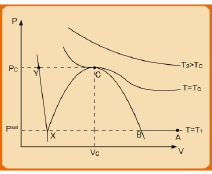(a)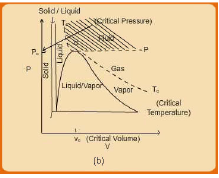(b)

Fig. 2.1 General P-V plots for real fluids

pressurization more of the saturated vapour present at B progressively liquefies until a point X is reached where all the original gas (or saturated vapour) is fully converted to liquid state. Point X is described as a saturated liquid state. It follows that at all point between B and X the substance exists partitioned into two phases, i.e., part vapour and part liquid. As one transits from B to X, pressure and temperature both remain constant; the only change that occurs is that the fraction of the original gas at point A (or B) that is liquefied increases, until it is 1.0 at point X. The line B-X connecting the saturated vapour and liquid phases is called the tie-line. For a given T and P, the relative amounts of the phases determine the effective molar (or specific) volume at any point within the two-phase region. Any further attempt to pressurize the saturated liquid results in relatively very little compression, and this is captured by the steep slope of the curve X-Y, which signifies that the liquid state is far less compressible, compared to the gas state (i.e., points over A-B). Essentially points between X-Y (including Y itself) represent compressed liquid states.

An important point to re-emphasize is that on the two-phase line B-X, the pressure of the system remains constant at a fixed value. This pressure is termed the saturation pressure ( Psat ) corresponding to the temperature T1. We recall your attention to the phase rule described in section 1.5, and eqn. 1.11.  By this eqn. the degrees of freedom is one, which is borne by the fact that if one fixes temperature the system pressure also becomes fixed. However, in both regions AB and X-Y the degrees of freedom is two, as pressure becomes fixed only if one defines both temperature and volume.

In general, the same behviour as detailed above may repeat at another temperature T (>T1). One can on the one hand connect all the saturated vapour phase points at different temperatures and on the other connect all the points representing saturated liquid phase, the locus of such points give rise to the dome-shaped portion X-C-B of the P-V diagram which essentially signifies that at any pressure and volume combination within this dome, the state of the system is biphasic (part gas and part liquid). The region right of the dome B-C represents saturated gas phase while to the left (X-C) the state is saturated liquid. If one continues to conduct the pressurization at increasingly higher temperatures, one eventually arrives at a temperature for which the tie-line is reduced to a point and the P-V curve turns into an inflexion point to the twophase dome. The temperature which such a behavior obtains is called the critical temperature (TC), while the pressure at corresponding point of inflexion is termed the critical pressure (PC).

The molar volume at the point is termed the critical volume, and the state itself the critical point. A fluid which is at a temperature and pressure above the critical point values is said to be in a supercritical state; this is indicated by the hatched region in fig. 2b. As has been shown for the PV curves for a T > TC
Fig. 2.2 Pressure-Temperature Diagram of a Pure Substance, there exists no liquid phase as the curve passes beyond the two-phase dome region. Thus, the critical temperature is a temperature above which a gas cannot be liquefied by compressing, as can be below it. Compilation of values of critical properties and ω for a large number of substances are available readily from several sources.

In fig. 2.1b the phase behavior depicted in fig. 2.1a is extended and more generalized to include solid phase as well. Accordingly, not only vapour-liquid region, other two phase regions, i.e., solid-vapour and solid-liquid regions are also displayed. The same arguments as made above for explaining the nature of co-existence of vapour and liquid phases apply to the other two biphasic regions.

P-T Diagrams

The phase behaviour described by fig. 2.1 can also be expressed in a more condensed manner by means of a pressure-temperature (P-T) diagram shown in fig 2.2. Just as P-V curves were depicted at constant temperature, the P-T diagram is obtained at a constant molar volume. The two phase regions which were areas in the P-V diagram are reduced to lines (or curves) in fig. 2.2. The P-T curves shown by lines X-Y, Y-Z, and Y-C result from measurements of the vapour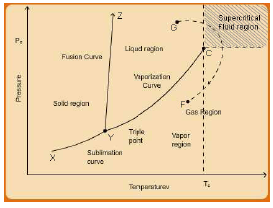Fig. 2.2 Pressure-Temperature Diagram of a Pure Substance

pressure of a pure substance, both as a solid and as a liquid. X-Y corresponds to the solid-vapour (sublimation) line; X-Y represents the co-existence of solid and liquid phases or the fusion line, while the curve Y-C displays the vapour-liquid equilibrium region. The pressure at each temperature on the Y-C curve corresponds to the equilibrium vapour pressure. (Similar considerations apply for P-T relation on the sublimation curve, X-Y). The terminal point C represents the critical point, while the hatched region corresponds to the supercritical region. It is of interest to note that the above three curves meet at the triple-point where all three phases, solid, liquid and vapour co-exist in equilibrium. By the phase rule (eqn. 1.11) the degrees of freedom at this state is zero. It may be noted that the triple point converts to a line in fig. 2.1b. As already noted, the two phases become indistinguishable at the critical point. Paths such as F to G lead from the liquid region to the gas region without crossing a phase boundary. In contrast, paths which cross phase boundary Z-Y include a vaporization step, where a sudden change from liquid to gas occurs.

A substance in the compressed liquid state is also often termed as sub-cooled, while gas at a pressure lower than its saturation vapour pressure for a given temperature is said to be “superheated”. These descriptions may be understood with reference to fig. 2.2. Let us consider a compressed liquid at some temperature (T) and pressure (P). The saturation temperature for the pressure P would be expected to be above the given T. Hence the liquid is said to be sub-cooled with respect to its saturation temperature. Consider next a pure vapour at some temperature (T) and pressure (P). Clearly for the given pressure P the saturation temperature for the pressure P would be expected to be below the given T.  Hence with respect to the saturation temperature the vapour is superheated.

The considerations for P-V and P-T diagrams may be extended to describe the complete P-V-T phase behaviour in the form of three dimensional diagrams as shown in fig. 2.3. Instead of two-dimensional plots in figs. 2.1 and 2.2 we obtain a P-V-T surface. The P-V plots are recovered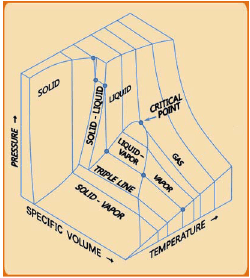Fig. 2.3 Generalized Three-dimensional P-V-T Surface for a Pure Substance

if one takes a slice of the three dimensional surface for a given temperature, while the P-T curve obtains if one takes a cross-section at a fixed volume. As may be evident, depending on the volume at which the surface is cut the P-T diagram changes shape.
Fig. 2.4 illustrates the phase diagram for the specific case of water. The data that is pictorially depicted so, is also available in the form of tables popularly known as the “steam table”.  The steam table  provides values of the following thermodynamic properties of water and vapour as a function of temperature and pressure starting from its normal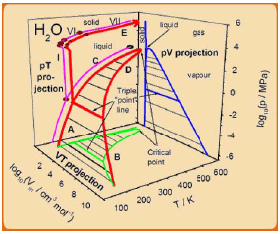Fig. 2.4 Three-dimensional P-V-T Plot for Water

freezing point to the critical point: molar volume, internal energy, enthalpy and entropy.

The steam tables are available for saturated (two-phase), the compressed liquid and superheated vapour state properties. The first table presents the properties of saturated gas and liquid as a function of temperature (and in addition provides the saturation pressure). For the other two states the property values are tabulated in individual tables in terms of temperature and pressure, as the degree of freedom is two for a pure component, single state. For fixing the values of internal energy, enthalpy and entropy at any temperature and pressure those for the saturated liquid state at the triple point are arbitrarily assigned zero value. The steam tables comprise the most comprehensive collection of properties for a pure substance.

Offer running on EduRev: Apply code STAYHOME200 to get INR 200 off on our premium plan EduRev Infinity!

## Thermodynamics

56 videos|92 docs|33 tests

,

,

,

,

,

,

,

,

,

,

,

,

,

,

,

,

,

,

,

,

,

;